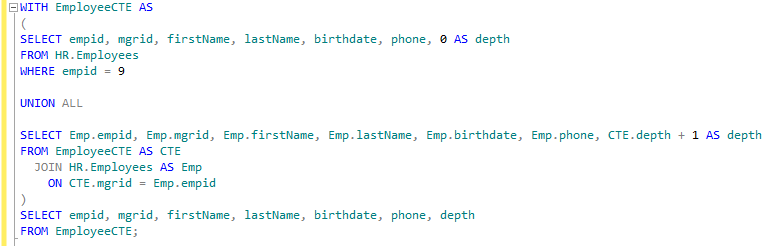CTE Recursion in Sql Server

1. This page will show you how to implement a recursive CTE.

Setting Up The Outer Query

1. The outer will be the one that will return us our final recursion results. Set it up as follows, we will fill in the parenthesis in the next section.
Substitute your own variables inside the brackets.

WITH [CTE Table Name] AS
(
[Inner Query's]
)
SELECT [table variables]
From [CTE Table];

Setting Up The Inner Query's

1. The inner query will contain 2 seprate queries connected by a UNION ALL. The first query

2. The first query will set the starting point for our recursion and shoukd be set up as follows. Substitute your own variables inside the brackets.
Be sure to include a variable that you can increment with. (i.e: 0 AS depth)

SELECT [Table Variables], 0 AS [Increment Variable]
FROM [Source Table]
WHERE [Condition for Selection]

3. Next we will add the UNION ALL and the second query below the first query.

4. The second query will be the one to do the recursion.

SELECT [Table Variables], 0 AS [Increment Variable]
FROM [Source Table]
WHERE [Condition for Selection]

UNION ALL

SELECT [Temp Two].[Table Variables], [Temp One].[Increment Variable] + 1 AS [Increment Variable]
FROM [CTE Table Name] AS [Temp One]
JOIN [Source Table] AS [Temp Two]
ON [Temp One].[Key] = [Temp Two].[Key]

Final Results

1. Once you are done, your code should look like this:

WITH [CTE Table Name] AS
(
SELECT [Table Variables], 0 AS [Increment Variable]
FROM [Table Name]
WHERE [Condition for Selection]

UNION ALL

SELECT [Temp Two].[Table Variables], [Temp One].[Increment Variable] + 1 AS [Increment Variable]
FROM [CTE Table Name] AS [Temp One]
JOIN [Source Table] AS [Temp Two]
ON [Temp One].[Key] = [Temp Two].[Key]
)
SELECT [table variables]
From [CTE Table];

2.